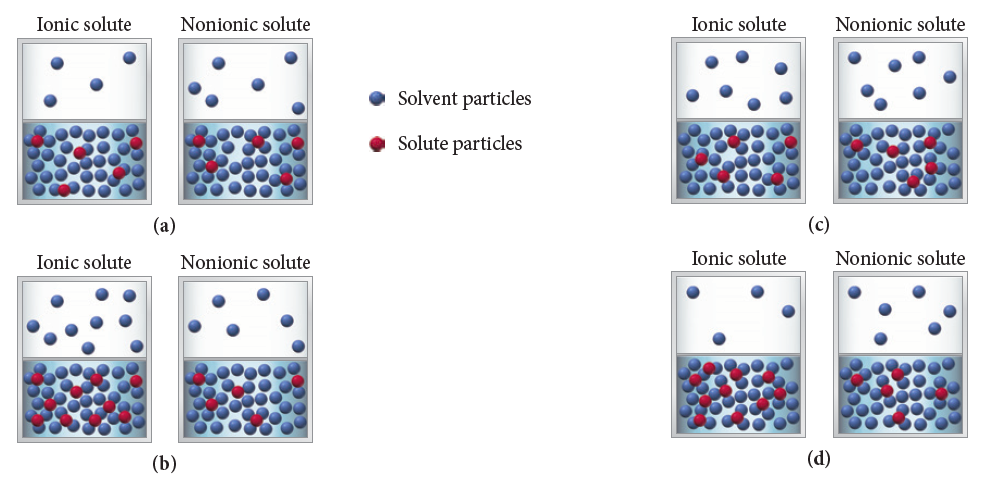# Problem: The vapor pressure of a 1 M ionic solution is different from the vapor pressure of a 1 M nonionic solution. In both cases, the solute is nonvolatile. Which of the following sets of diagrams best represents the differences between the two solutions and their vapors?

###### FREE Expert Solution

Vapor Pressure is achieved by the equilibrium rate of vaporization equaling the rate of condensation.

An ionic solution would have a lower vapor pressure as compared to a non-ionic solution. Recall that we have both have the same nonvolatile solute.

The appropriate diagram therefore is:

79% (122 ratings)###### Problem Details

The vapor pressure of a 1 M ionic solution is different from the vapor pressure of a 1 M nonionic solution. In both cases, the solute is nonvolatile. Which of the following sets of diagrams best represents the differences between the two solutions and their vapors?Frequently Asked Questions

What scientific concept do you need to know in order to solve this problem?

Our tutors have indicated that to solve this problem you will need to apply the The Colligative Properties concept. You can view video lessons to learn The Colligative Properties. Or if you need more The Colligative Properties practice, you can also practice The Colligative Properties practice problems.

What professor is this problem relevant for?

Based on our data, we think this problem is relevant for Professor Frasso's class at WVU.